## If the first-order maximum for monochromatic light falling on a double slit is at an angle of 10.0∘, at what angle is the second-order maxim

Question

If the first-order maximum for monochromatic light falling on a double slit is at an angle of 10.0∘, at what angle is the second-order maximum?

in progress 0
2 months 2021-08-01T01:05:19+00:00 1 Answers 6 views 0

The  value is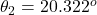Explanation:

From the question we are told that

The angle of the first order maximum is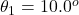Generally the condition for constructive interference is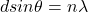Here  d is the separation between the slit ,

n  is the order of maxima  with values n  =  1, 2 , 3 … for first , second , third … order of maxima

Now for first order of maximum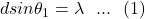=>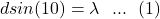Now for second order of maximum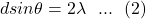dividing equation 1  by 2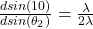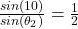=>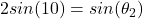=>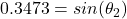=>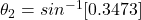=>